# Texas Go Math Grade 6 Lesson 2.3 Answer Key Comparing and Ordering Rational Numbers

Refer to our Texas Go Math Grade 6 Answer Key Pdf to score good marks in the exams. Test yourself by practicing the problems from Texas Go Math Grade 6 Lesson 2.3 Answer Key Comparing and Ordering Rational Numbers.

## Texas Go Math Grade 6 Lesson 2.3 Answer Key Comparing and Ordering Rational Numbers

Reflect

Question 1.
Communicate Mathematical Ideas How does a number line represent equivalent fractions and decimals?
The number line represents equivalent fractions and decimals as same exact points on it!

Question 2.
Name a decimal between 0.4 and 0.5.
Decimal between 0.4 and 0.5 would be any number that is greater than 0.4 and smaller than 0.5.

Now, we know that our decimal could be 0.45 or even 0.46754. We could pick any decimal which value is between 0.4 and 0.5

Order the fractions and decimals from least to greatest.

Question 3.
0.85, $$\frac{3}{5}$$, 0.15, $$\frac{7}{10}$$
First thing will be writing decimals as equivalent fractions!Second thing will be finding common denominator for our fractions!
Let’s get all the numbers to have 20 as common denominator!
We multiply both, numerator and denominator with same number to get equivalent expression!
$$\frac{3}{5}=\frac{3 \cdot 4}{5 \cdot 4}$$ = $$\frac{12}{20}$$
$$\frac{7}{10}=\frac{7 \cdot 2}{10 \cdot 2}$$ = $$\frac{14}{20}$$

Now we compare numerators of fractions!
17, 3, 12, 14
So, now we know that 3 is smaller than 12 which is smaller than 14 which is smaller than 17 .
Now we can write them as: 3 < 12 < 11 < 17
Therefore:
$$\frac{3}{20}<\frac{12}{20}<\frac{14}{20}<\frac{17}{20}$$

Last thing that we want to do is to return expressions to given ones!
So, we have:
0.15 < $$\frac{3}{5}$$ < $$\frac{7}{10}$$ < 0.85
So, now we have ordered them from Least to greatest and we can write the order as:
0.15, $$\frac{3}{5}$$, $$\frac{7}{10}$$, 0.85

Reflect

Question 4.
Communicate Mathematical Ideas Describe a different way to order the numbers.
Different way to order the numbers would be:
Firstly, write them all as fractions!
Secondly, find equivalent fractions with common denominator!
Thirdly, compare numerators and list them from greatest to least.

Question 5.
To compare their bike times, the friends created a table that shows the difference between each person’s time and the average bike time. Order the bike times from least to greatest.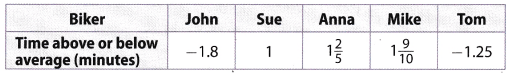First thing will be writing fractions as equivalent decimals!Now we use number line to conclude relation between numbers!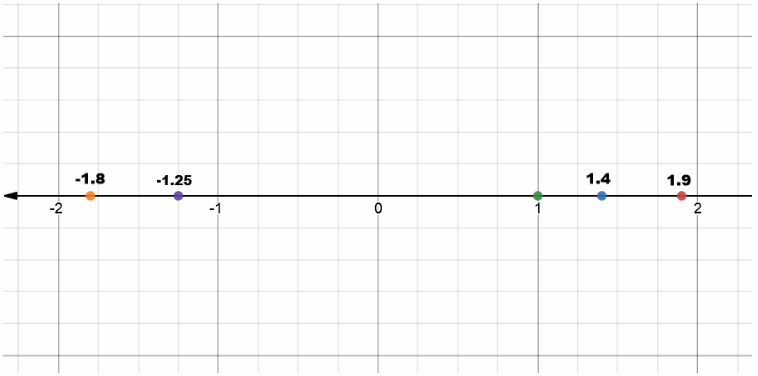Now, we know that:
Number – 1.8 is smaller than – 1.25 which is smaller than 1 which is smaller than 1.4 which is smaller than 1.9.
We can write it as:
– 1.8 < – 1.25 < 1 < 1.4 < 4.9

One last thing to do is to return decimals to given expressions and write it ¡n order from least to greatest!
– 1.8, – 1.25, 1, 1$$\frac{2}{5}$$, 1$$\frac{9}{10}$$

Find the equivalent fraction or decimal for each number.

Question 1.
0.6 = __________
Here, we want to find equivalent fraction to number 0.6
Firstly, we write it as fraction!
Secondly, we simplify it by dividing with greatest common divisor!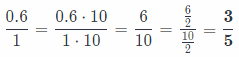Question 2.
$$\frac{1}{4}$$ = __________
Here, we want to write fraction $$\frac{1}{4}$$ as equivalent decimal number!
We divide numbers 1 and 4 to get our solution!$$\frac{1}{4}$$ = 0.25

Question 3.
0.9 = __________
Here, we want to find equivalent fraction to number 0.9
Firstly, we write it as fraction!
Secondly, we simplify it by dividing with greatest common divisor, only if we can!
0.9 = $$\frac{0.9 \cdot 10}{1 \cdot 10}=\frac{\mathbf{9}}{\mathbf{1 0}}$$

Question 4.
0.1 = __________
Here, we want to find equivalent fraction to number 0.1
Firstly, we write it as fraction!
Secondly, we simplify it by dividing with greatest common divisor, only if we can!
0.1 = $$\frac{0.1 \cdot 10}{1 \cdot 10}=\frac{1}{10}$$

Question 5.
$$\frac{3}{10}$$
Here, we want to write fraction $$\frac{3}{10}$$ as equivalent decimal number!
We divide numbers 3 and 10 to get our solution!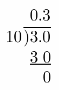$$\frac{3}{10}$$ = 0.3

Question 6.
1.4 = __________
Here, we want to find equivalent fraction to number 1.4
Firstly, we write it as fraction!
Secondly, we simplify it by dividing with greatest common divisor!Question 7.
$$\frac{4}{5}$$
Here, we want to write fraction $$\frac{4}{5}$$ as equivalent decimal number!
We divide numbers 4 and 5 to get our solution!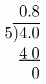$$\frac{4}{5}$$ = 0.8

Question 8.
0.4 = __________
Here, we want to find equivalent fraction to number 0.4
Firstly, we write it as fraction!
Secondly, we simplify it by dividing with greatest common divisor!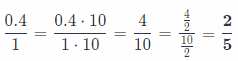Question 9.
$$\frac{6}{8}$$ = __________
Here, we want to write fraction $$\frac{6}{8}$$ as equivalent decimal number!
We divide numbers 6 and 8 to get our solution!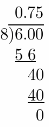$$\frac{6}{8}$$ = 0.75

Use the number line to order the fractions and decimals from least to greatest.

Question 10.
0.75, $$\frac{1}{2}$$, 0.4, and $$\frac{1}{5}$$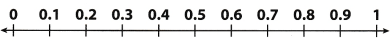First thing will be writing fractions as equivalent decimals!
$$\frac{1}{2}$$ = 0.5
$$\frac{1}{5}$$ = 0.2Now we know that number 0.25 is smaller than 0.4 which is smaller than 0.5 which is smaller than 0.75.
One last thing to do is to return decimals to given expressions and write it in order from least to greatest!
$$\frac{1}{5}$$, 0.4, $$\frac{1}{2}$$, 0.75

Question 11.
The table shows the lengths of fish caught by three friends at the lake last weekend. Write the lengths in order from greatest to least.First thing will be writing fractions as equivalent decimals!
12$$\frac{3}{5}$$ = 12 + $$\frac{3}{5}$$ = 12 + 0.6 = 12.6
12$$\frac{3}{4}$$ = 12 + $$\frac{3}{4}$$ = 12 + 0.75 = 12.75

Now we know that number 12.75 is greater than 12.7 which is greater than 12.6 .
One last thing to do is to return decimals to given expressions and write it in order from greatest to least!
12$$\frac{3}{5}$$, 12.7, 12$$\frac{3}{4}$$

Like the fractions and decimals in order from least to greatest.

Question 12.
2.3, 2$$\frac{4}{5}$$, 2.6
First thing will be writing fractions as equivalent decimals!
2$$\frac{4}{5}$$ = 2 + $$\frac{4}{5}$$ = 2 + 0.8 = 2.8

Now we know that number 2.3 is smaller than 2.6 which is smaller than 2.8.
One Last thing to do is to return decimals to given expressions and write it in order from greatest to least!
2.3, 2.6, 2$$\frac{4}{5}$$

Question 13.
0.5, $$\frac{3}{16}$$, 0.75, $$\frac{5}{48}$$
First thing will be writing fractions as equivalent decimals!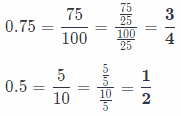Second thing will be finding common denominator for our fractions!
Let’s get all the numbers to have 48 as common denominator!
We multiply both, numerator and denominator with same number to get equivalent expression!
$$\frac{3}{4}$$ = $$\frac{3 \cdot 12}{4 \cdot 12}=\frac{36}{48}$$
$$\frac{1}{2}$$ = $$\frac{1 \cdot 24}{2 \cdot 24}=\frac{24}{48}$$
$$\frac{3}{16}$$ = $$\frac{3 \cdot 3}{16 \cdot 3}=\frac{9}{48}$$

Now we compare numerators of fractions!
5, 36, 24, 9
So, now we know that 5 is smaller than 9
which is smaller than 14 which is smaller than 17 .
Now we can write them as : 5 < 9 < 24 < 36
Therefore:
$$\frac{5}{48}<\frac{9}{48}<\frac{24}{48}<\frac{36}{48}$$

Last thing that we want to do is to return expressions to given ones!
So, we have:
$$\frac{5}{48}$$ < $$\frac{3}{16}$$ < 0.5 < 0.75
So, now we have ordered them from least to greatest and we can write the order as:
$$\frac{5}{48}$$, $$\frac{3}{16}$$, 0.5, 0.75

Question 14.
0.5, $$\frac{1}{5}$$, 0.35, $$\frac{12}{25}$$, $$\frac{4}{5}$$
First thing will be writing fractions as equivalent decimals!
0.35 = $$\frac{35}{100}$$
0.5 = $$\frac{5}{10}=\frac{5 \cdot 10}{10 \cdot 10}=\frac{50}{100}$$

Second thing will be finding common denominator for our fractions!
Let’s get all the numbers to have 100 as common denominator!
We multiply both, numerator and denominator with same number to get equivalent expression!
$$\frac{4}{5}$$ = $$\frac{4 \cdot 20}{5 \cdot 20}=\frac{80}{100}$$
$$\frac{12}{25}$$ = $$\frac{12 \cdot 4}{25 \cdot 4}=\frac{48}{100}$$
$$\frac{1}{5}$$ = $$\frac{1 \cdot 20}{5 \cdot 20}=\frac{20}{100}$$

Now we compare numerators of fractions!
20, 48, 80, 35, 50
So, now we know that 20 is smaller than 35
which is smaller than 48 which is smaller than 50 which is smaller than 80
Now we can write them as : 20 < 35 < 48 < 50 < 80
Therefore:
$$\frac{20}{100}<\frac{35}{100}<\frac{48}{100}<\frac{50}{100}<\frac{80}{100}$$

Last thing that we want to do is to return expressions to given ones!
So, we have:
$$\frac{1}{5}$$ < 0.35 < $$\frac{12}{25}$$ < $$\frac{1}{2}$$ < $$\frac{4}{5}$$
So, now we have ordered them from least to greatest and we can write the order as:
$$\frac{1}{5}$$, 0.35, $$\frac{12}{25}$$, $$\frac{1}{2}$$, $$\frac{4}{5}$$

Question 15.
$$\frac{3}{4},-\frac{7}{10},-\frac{3}{4}, \frac{8}{10}$$
First thing will be writing decimals as equivalent fractions!
Since they are all given by fractions, we can skip this step!

Second thing will be finding common denominator for our fractions!
Let’s get all the numbers to have 20 as common denominator!
We multiply both, numerator and denominator with same number to get equivalent expression!Now we compare numerators of fractions!
– 14, 15, 16, – 15
So, now we know that – 15 is smaller than – 14
which is smaller than 15 which is smaller than 16
Now we can write them as : – 15 < – 14 < 15 < 16
Therefore:
$$\frac{-15}{20}<\frac{-14}{20}<\frac{15}{20}<\frac{16}{20}$$

Last thing that we want to do is to return expressions to given ones!
So, we have:
– $$\frac{3}{4}$$ < – $$\frac{7}{10}$$ < $$\frac{3}{4}$$ < $$\frac{8}{10}$$
So, now we have ordered them from least to greatest and we can write the order as:
– $$\frac{3}{4}$$, – $$\frac{7}{10}$$, $$\frac{3}{4}$$, $$\frac{8}{10}$$

Question 16.
– $$\frac{3}{8}$$, $$\frac{5}{16}$$, – 0.65, $$\frac{2}{4}$$
First thing will be writing fractions as equivalent decimals!
Note that i will do division all the way down!
– $$\frac{3}{8}$$ = – 0.375
$$\frac{5}{16}$$ = 0.3125
$$\frac{2}{4}$$ = $$\frac{1}{2}$$ = 0.5

Now we know that number – 0.65 is smaLler than – 0.375 which is smaller than 0.3125 which is smaller than 0.5.
One last thing to do is to return decimals to given expressions and write it in order from least to greatest!
– 0.65, – $$\frac{3}{8}$$, $$\frac{5}{16}$$, $$\frac{2}{4}$$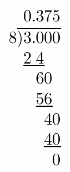Question 17.
– 2.3, – 2$$\frac{4}{5}$$, – 2.6
First thing will be writing fractions as equivalent decimals!
– 2$$\frac{4}{5}$$ = – 2 – $$\frac{4}{5}$$ = – 2 – 0.8 = – 2.8

Now we know that number – 2.8 is smaller than – 2.6 which is smaller than – 2.3.
One last thing to do is to return decimals to given expressions and write it in order from greatest to least!
– 2$$\frac{4}{5}$$, – 2.6, – 2.3

Question 18.
– 0.6, – $$\frac{5}{8}$$, – $$\frac{7}{12}$$, – 0.72
First thing will be writing fractions as equivalent decimals!
Note that i will do division all the way down!
– $$\frac{5}{8}$$ = – 0.625
– $$\frac{7}{12}$$ = – 0.58$$\dot{3}$$

Now we know that number – 0.72 is smaller than – 0.625 which is smaller than – 0.6 which is smaller than – 0.58$$\dot{3}$$.
One last thing to do is to return decimals to given expressions and write it in order from least to greatest!
– 0.72, –$$\frac{5}{8}$$, – 0.6, – $$\frac{7}{12}$$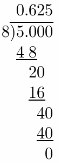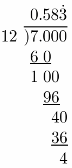Question 19.
1.45, 1$$\frac{1}{2}$$, 1$$\frac{1}{3}$$, 1.2
First thing will be writing fractions as equivalent decimals!
1$$\frac{1}{2}$$ = 1 + $$\frac{1}{2}$$ = 1 + 0.5 = 1.5
1$$\frac{1}{3}$$ = 1 + $$\frac{1}{3}$$ = 1 + 0.3 = 1.$$\dot{3}$$
Note that i will write division all the way down!

Now we know that number 1.2 is smaller than 1.$$\dot{3}$$ which is smaller than 1.45 which is smaller than 1.5
One last thing to do is to return decimals to given expressions and write it in order from greatest to least!
1$$\frac{1}{2}$$, 1$$\frac{1}{3}$$, 1.45, 1.5Question 20.
– 0.3, 0.5, 0.55, – 0.35
First thing will be writing fractions as equivalent decimals!
Since all the numbers are written in appropriate form, we can proceed to next step!

Now we know that number – 0.35 is smaLler than – 0.3 which is smaller than 0.5 which is smaller than 0.55.
One last thing to do is to return decimals to given expressions and write it in order from least to greatest!
– 0.35, – 0.3,0.5, 0.55

Question 21.
Explain how to compare 0.7 and $$\frac{5}{8}$$
we can solve this problem it two Ways.
As given, 0.7 is already written as decimal and therefore easiest way would include dividing number 5 with 8 to transform it into decimal.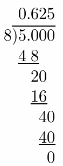Now, when we have both number as decimals, we can simply conclude that 0.7 is greater than 0.625.
So, we can write:
0.7 > $$\frac{5}{8}$$

Question 22.
Rosa and Albert receive the same amount of allowance each week. The table shows what part of their allowance they each spent on video games and pizza. Use a number line to help you compare.a. Who spent more of their allowance on video games? Write an inequality to compare the portion spent on video games.
We know that Rosa spent 0.4, and Albert $$\frac{1}{2}$$ of their allowance on video games.
We can convert $$\frac{1}{2}$$ into 0.5 by dividing 1 with 2.
Now, we can conclude that 0.5 is greater than 0.4, and we can write:
0.5 > 0.4
So Albert spent more of his allowance than Rosa on video games.

b. Who spent more of their allowance on pizza? Write an inequality to compare the portion spent on pizza.
We know that Rosa spent $$\frac{2}{5}$$, and Albert 0.25 of their allowance on pizza.
We can convert $$\frac{2}{5}$$ into 0.4 by dividing 2 with 5.
Now, we can conclude that 0.4 is greater than 0.25, and we can write:
0.4 > 0.25
So Rosa spent more of her allowance than Albert on pizza.

c. Draw Conclusions Who spent the greater part of their total allowance? How do you know?
All we need to do here is to add allowance values for each of kids, and compare them!
So we have:
0.4 + 0.4 = 0.8
0.5 + 0.25 = 0.75
Now we can conclude that 0.8 is greater than 0.75 and therefore we can say:
Rosa spent more of her allowance than Albert did!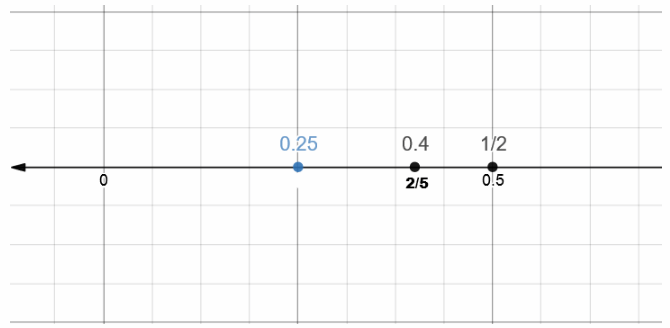Question 23.
A group of friends is collecting aluminum for a recycling drive. Each person who donates at least 4.25 pounds of aluminum receives a free movie coupon. The weight of each person’s donation is shown in the table.a. Order the weights of the donations from greatest to least.
Firstly, we will transform all fractions to decimals!
So, we have:
6$$\frac{1}{6}$$ = 6 + $$\frac{1}{6}$$ = $$\frac{37}{6}$$ = 6.1$$\dot{6}$$
$$\frac{15}{4}$$ = 3.75
4$$\frac{3}{8}$$ = 4 + $$\frac{3}{8}$$ = $$\frac{35}{8}$$ = 4.375
Now, we can compare those donations!
Number 6.1$$\dot{6}$$ is greater than 5.5 which is greater than 4.375 which is greater than 4.3 which is greater than 3.75
Now we can return numbers to its original forms and write them in order from greatest to least:
6$$\frac{1}{6}$$, 5.5, 4$$\frac{3}{8}$$, 4.3, $$\frac{15}{4}$$

b. Which of the friends will receive a free movie coupon? Which will not?
We know that only students who donated more than 4.25 pounds of aluminum will receive a free movie coupon.
Therefore, we will compare given values with 4.25 and conclude which of students is not going to get a free
movie coupon.
We can compare each number with 4.25 but it is not necessary because we ordered them from greatest to least in
Therefore, we go from right side and compare numbers until we reach one which is greater than 4.25.
$$\frac{15}{4}$$ = 3.75
3.75 is smaller than 4.25, so Micah won’t receive the free movie coupon!
4.3 is greater than 4.25, so Brenda will receive free movie coupon!
Now we know that every other student will receive free movie coupon as their donations are higher!
So the following people will receive free movie coupon: Jim, Peter Brenda, Claire, and Micah won’t.

c. What If? Would the person with the smallest donation win a movie coupon if he or she had collected $$\frac{1}{2}$$ pound more of aluminum? Explain.
To find out would Micah receive free movie coupon if he had additional $$\frac{1}{2}$$ pounds of aluminum, we will have to add these values and compare its sum to 4.25
$$\frac{15}{4}+\frac{1}{2}=\frac{15}{4}+\frac{1 \cdot 2}{2 \cdot 2}=\frac{15}{4}+\frac{2}{4}=\frac{17}{4}$$
Now we transform this into decimal number and get:
$$\frac{17}{4}$$ = 4.25
Now we know that Micah’s new donation wilt be 4.25 pounds of aluminum
Since every person who donates at least 4.25 pounds of aluminum receives free movie coupon, Micah will receive it!

Division: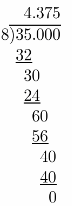Question 24.
Last week, several gas stations in a neighborhood all charged the same price for a gallon of gas. The table below shows how much gas prices have changed from last week to this week.a. Order the numbers in the table from least to greatest.
Firstly, we will transform all fractions to decimals!
So, we have:Now, we can compare those gas prices!
Number – 6.75 is smaller than – 6.6 which is smaller than – 5.625 which is smaller than 5.4 which is smaller than 5.8.
Now we can return numbers to its original forms and write them in order from greatest to least:
– 6$$\frac{3}{4}$$, – 6.6, – 5$$\frac{5}{8}$$, 5.4, 5.8

b. Which gas station has the cheapest gas this week?
As we ordered them from least to greatest in last task, we know that the gas price is lowest in Gas and Go at price change of – 6$$\frac{3}{4}$$

c. Critical Thinking Which gas station changed their price the least this week?
To find out, which gas station changed their gas price the least this weeks we can calculate absolute value of each price and figure which is lowest.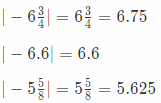As absolute value will give same result for positive numbers, We won’t include them in calculations.
We can draw these values on number line to see their relation, on simply compare them:
Number 6.75 is greater than 6.6 which is greater than 5.8 which is greater than 5.625 which is greater than 5.4.
Therefore, we can say that:
$$\frac{27}{5}$$ or 5.4 is the smallest value!
So, least change is made in Corner Store

Division: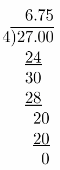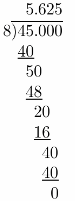H.O.T. Focus on Higher Order Thinking

Question 25.
Analyze Relationships Explain how you would order from least to greatest three numbers that include a positive number, a negative number, and zero.
It is actually very Simple. If we order them from least to greatest, negative number is always least, zero is in middle and positive number is greatest.
We can always draw them on number Line to convince our selves!

Question 26.
Critique Reasoning Luke is making pancakes. The recipe calls for 0.5 quart of milk and 2.5 cups of flour. He has $$\frac{3}{8}$$ quart of milk and $$\frac{18}{8}$$ cups of flour. Luke makes the recipe with the milk and flour that he has. Explain his error.
Luke hasn’t got enough milk and cups of flour
Let’s prove that!
Firstly, we transform given fractions into decimals.
$$\frac{3}{8}$$ = 0.375
$$\frac{18}{8}$$ = 2.25
Since 0.375 is less than 0.5 and 2.25 is less than 2.5.
We can conclude that Luke is missing both milk and cups of flour!

Division: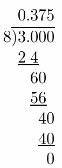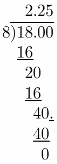Question 27.
Communicate Mathematical Ideas If you know the order from least to greatest of 5 negative rational numbers, how can you use that information to order the absolute values of those numbers from least to greatest? Explain.